# Binding

In this chapter you will learn the basics of binding and how we can execute code in different execution contexts.

## Background

We have already covered the basic concepts of variables, methods and self. This chapter will combine all those concepts into one.## What is Binding?

A Binding object encapsulates the execution context at a particular place in the program. The execution context consists of the variables, methods and value of self. This context can be later accessed via the built-in function binding. We can create the binding object by using Kernel#binding method. The Kernel#eval method takes binding object as the second argument. Thus, the binding object can establish an environment for code evaluation.

## The Execution Context

In The main Object chapter, you saw this diagram: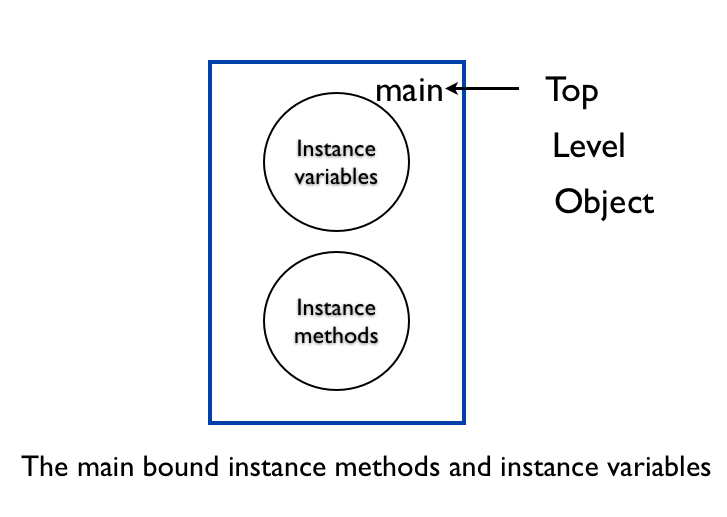The particular place in the program in this example is the top level. The value of self is main. We also saw how the instance variables and the instance methods are bound to the main object. The above diagram is the execution context for the top level. The diagram can be redrawn to make the binding concept clear.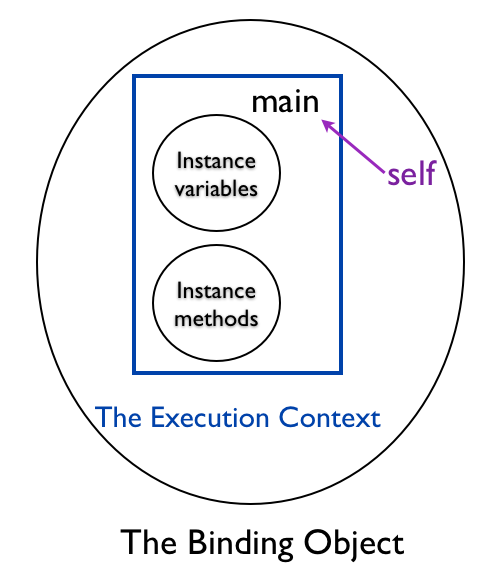Why do we need Binding?

Code does not run in a vacuum. Code combined with an execution context becomes a running program.### Skeleton Analogy

Code is like a skeleton.Execution Context is like the human flesh and skin.Just like the human flesh and skin on a skeleton forms the human body. Running program is the combination of code and execution context.

### Self at the Top Level

We can verify that the binding object at the top level context has main as the value of self.

``````p TOPLEVEL_BINDING.receiver
``````

This prints:

``````main
``````

The TOPLEVEL_BINDING is a Ruby built-in constant that captures the binding at the top level.### Local Variables at the Top Level

Let's check for local variables defined at the top level.

``````p TOPLEVEL_BINDING.local_variables
``````

This prints an empty array.

``````[]
``````

Let's define a local variable at the top level.

``````p TOPLEVEL_BINDING.local_variables
x = 1
``````

This prints :

``````[:x]
``````

Ruby read all the statements at the top level, so it was able to print the value of x that comes even after the print statement.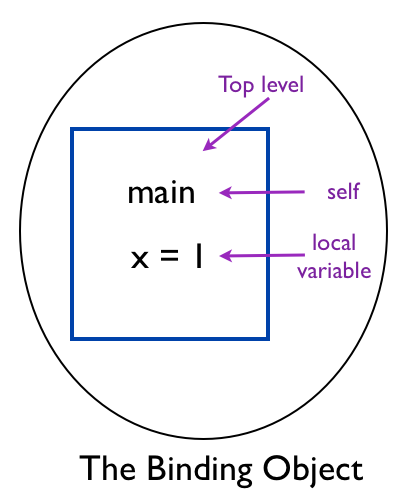### Instance Variable at the Top Level

How can we inspect the instance variable at the top level in the binding object?

``````@y = 0
p TOPLEVEL_BINDING.instance_variables
``````

This prints an empty array.

``````[]
``````

However, if we set the instance variable dynamically, we can print it.

``````TOPLEVEL_BINDING.instance_variable_set('@y', 0)
p TOPLEVEL_BINDING.instance_variables
``````

This prints:

``````[:@y]
``````

We can also read the value of the instance variable at the top level.

``````TOPLEVEL_BINDING.instance_variable_set('@y', 0)
p TOPLEVEL_BINDING.instance_variable_get('@y')
``````

This prints:

``````0
``````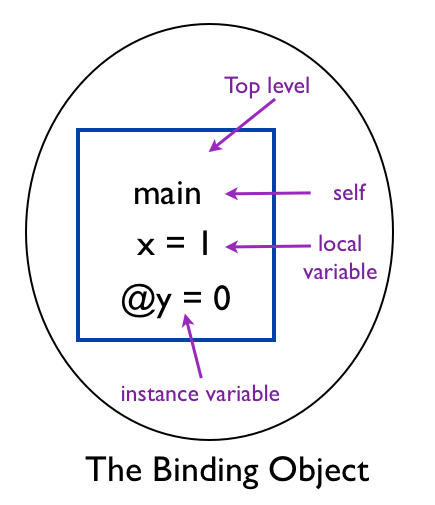### Accessing the Local Variable at the Top Level

Let's use eval to access the local variable at the top level.

``````binding_before_x = binding
p "Before defining x : #{eval("x", binding_before_x)}"

x = 1

binding_after_x = binding
p "After defining x : #{eval("x", binding_after_x)}"
``````

This prints:

``````Before defining x :
After defining x : 1
``````

The local variable x did not have any value before the assignment statement.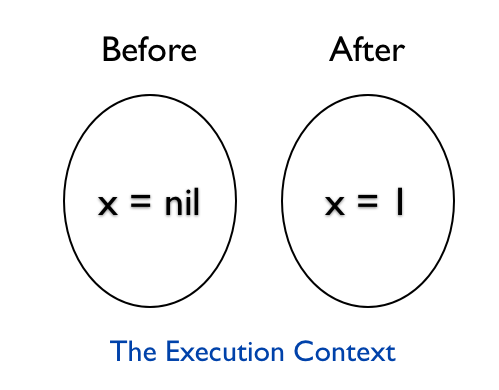You can see the difference in this program from the previous section Instance Variable at the Top Level. The eval evaluates the value of x at the top level at the point at which it encounters. We then print the value before and after the local variable is initialized.

## Object Context

### Finding the self using Binding Object

Here is the example we saw in Same Sender and Receiver chapter.

``````class Car
def drive
p "self is : #{self}"
start
end

private
def start
p 'starting...'
end
end

c = Car.new
c.drive
``````

Let's rewrite the above example to use the binding object to find the receiver.

``````class Car
def drive
p "self is : #{self}"
start
end

private
def start
p 'starting...'
end
end

c = Car.new
c.drive
``````

This example uses:

``````binding.receiver
``````

to print the receiver object. The output is:

``````self is : #<Car:0x007fe373864dc8>
starting...
``````

The self and the receiver is the same car object.

Can I find the sender using the binding object?

No, binding object does not have a sender method that can give us the sender object.

### Accessing the Instance Variable

In What is an Object chapter we could not access the color instance variable of the car object.

``````class Car
def initialize(color)
@color = color
end

def drive
'driving'
end
end

car = Car.new('red')
p car.color
``````

This example gave the error:

``````NoMethodError: undefined method ‘color’ for #<Car:0x007f9be4025bc0 @color="red">
``````

How can we access the color instance variable in the car object using binding? We know eval method takes the code as the first argument and binding as the second argument. Let's print the result of eval that takes the color instance variable and binding of the car object.

``````class Car
def initialize(color)
@color = color
end

def drive
'driving'
end
end

car = Car.new('red')
p eval("@color", car.binding)
``````

This results in the error:

``````NoMethodError: private method ‘binding’ called for #<Car:0x007ffb639adbc8 @color="red">
``````

Kernel module defines the binding method. Thus, it is available as a private method in the Object. Let's define a my_binding method that will provide us access to the execution context.

``````class Car
def initialize(color)
@color = color
end

def drive
'driving'
end

def my_binding
binding
end
end

car = Car.new('red')
p eval("@color", car.my_binding)
``````

This prints:

``````red
``````

We are able to take a peek at the instance variable in the binding object. The binding object captures the value of self inside the my_binding method. We know that the value of self inside the my_binding method is a car object. The above example is the same as the following example:

``````class Car
def initialize(color)
@color = color
end

def drive
'driving'
end

def my_binding
puts @color
end
end

car = Car.new('red')
car.my_binding
``````

This also prints:

``````red
``````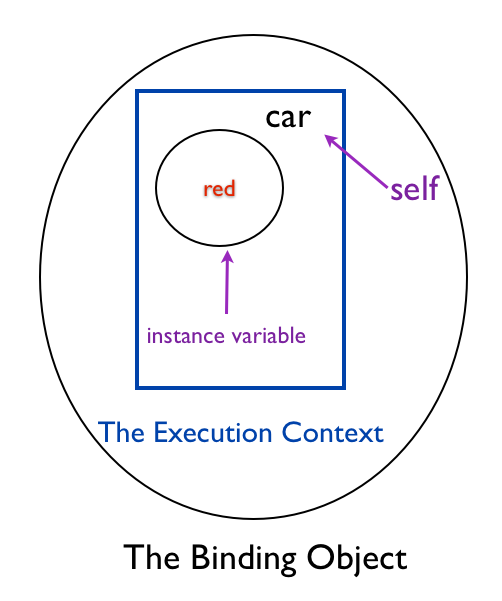We can verify the value of self by running the following example.

``````class Car
def initialize(color)
@color = color
end

def drive
return 'driving'
end

def my_binding
puts self
puts @color
end
end

car = Car.new('red')
car.my_binding
``````

This prints the car object memory location and the color.

``````#<Car:0x007fa132018e90>
red
``````

## Execution Context Analogy

It's like using a probe to send some piece of code to execute in a different context.## Executing Code in Different Execution Contexts

The power of binding is in the ability to run the same code in different contexts. Let's take a look at an example.

``````class Car
def initialize(color)
@color = color
end

def my_binding
binding
end
end

red_car = Car.new('red')
black_car = Car.new('black')

code = "@color"

p eval(code, red_car.my_binding)
p eval(code, black_car.my_binding)
``````

This prints:

``````red
black
``````There is no restriction on which object should provide the binding. We can have another class, let's say dog, that provides an execution context for the same code.

``````class Dog
def initialize(color)
@color = color
end

def my_binding
binding
end
end

dog = Dog.new('Brown')
code = "@color"
p eval(code, dog.my_binding)
``````

This prints:

``````Brown
``````

The my_binding method is like a probe. Code is put inside of this method.Why do we need the ability to run the code in different execution contexts?

Ruby is dynamic in nature. This allows us to reuse code in scenarios that we might not have imagined when we wrote the code.

## Executing Code in Different Scope

### Execute Code in an Object Scope from Top Level Scope

Let's look at an example that executes code in the rabbit object scope from the top level scope.

``````class Rabbit
def context
@first = 'Bugs'
last = 'Bunny'

binding
end
end

binding = Rabbit.new.context
# Scope here has changed because this is top level scope.
# But we are executing the following code in the rabbit object scope by using eval.
p eval("self", binding)
p eval("last.size", binding)
p eval('@first', binding)

# This uses binding only, no eval is used to get the local variable
p binding.local_variable_get('last')
``````

This prints:

``````#<Rabbit:0x007fc2a406c318 @first="Bugs">
5
Bugs
Bunny
``````

We were able to take a look at the local variable, instance variable, value of self and execute methods on the local variable. It is as if we were inside the context method like this:

``````class Rabbit
def context
@first = 'Bugs'
last = 'Bunny'

puts self
puts @first
puts last
puts last.size
end
end

Rabbit.new.context
``````

This prints:

``````#<Rabbit:0x007fe3f3881038>
Bugs
Bunny
5
``````### Execute Code in Top Level Scope from an Object Scope

Let's now see an example where we execute code in the top level scope when we are in an object scope.

``````@actor = 'Daffy'

class Actor
def self.act
eval("@actor", TOPLEVEL_BINDING)
end
end

p Actor.act
``````

This prints:

``````Daffy
``````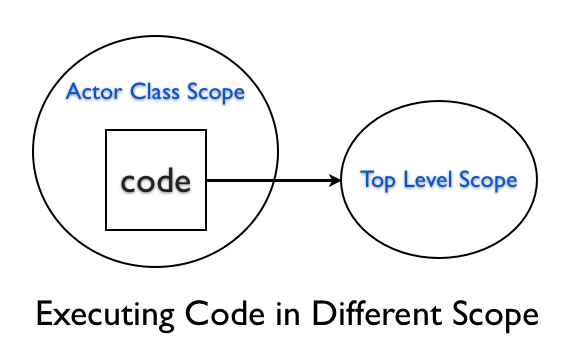If you access the top level instance variable inside the method from the Actor class scope, you will not be able to access the value.

``````@actor = 'Daffy'
class Actor
def self.act
@actor
end
end

p Actor.act
``````

This will print nil, because, the @actor inside the act method is in a different scope and it is not initialized. They are two different variables that happens to have the same name.

## Practical Example

Read the article Generate Documents using Templates. This example shows how we can apply what we have learned in this chapter to a real problem.

## Summary

In this chapter, we learned that we can package up the execution environment for later use via the binding. We looked at the value of self, local variable and the instance variable inside a binding object.